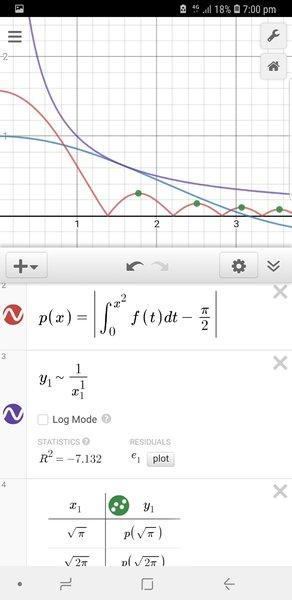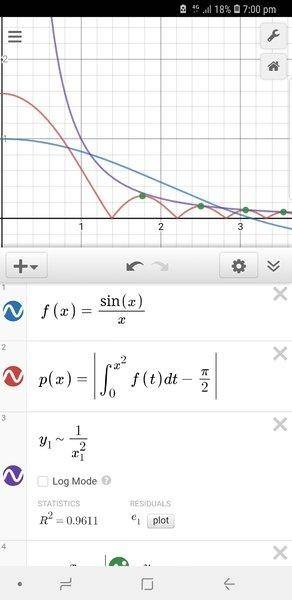# Area under a sine integral graph

• B
• Saracen Rue
In summary, the conversation discusses the convergence and divergence of the integral of the function ##Si(x^2)-\pi/2## as well as the use of Desmos app for graphing and modeling. The conversation also mentions the YouTube channel 3blue1brown as a resource for calculus and linear algebra. The conversation concludes with a suggestion to numerically calculate points for the sequence ##(a_j)_{j=1}^\infty## and ##(b_j)_{j=1}^\infty## related to the function's zero values and maximum values.

#### Saracen Rue

Hello,

I've recently discovered the sine integral and have been playing around with it a bit on some graphing software. I looked at the graph of ##Si(x^2) - \frac π 2## and saw that both the amplitude and period was decreasing as x increased. Curiosity got the best of me so I decided to calculate the integral of the function from 0 to infinity to find the area enclosed between the function and the x-axis, which turned out to be ##-\sqrt\frac π 2## square units. This makes sense due to the large portion of the graph being under the x-axis, however I was still curious to find out what the area unde the graph would be if the function was only above the x-axis so I tried integrating the absolute of the function. However here's where I had some trouble; nothing seems able to find the integral of this, not even Wolfram alpha (at least with standard computation time).

So long story short...

##\int_0^∞ Si(x^2) - \frac π 2 dx## = ##-\sqrt \frac π 2##

I want to find out what ##\int_0^∞ |Si(x^2) - \frac π 2 |dx## is equal to. I have tried using heaps of online integral calculators but none of them seem to be able to do it. Wolfram Alpha times out but I'm only a standard member, maybe someone with Pro can give it a go. My intuition tells me that the answer is going to be something like ##\sqrt π## but I'm really not sure if this is right or not.

Any help with this is greatly appreciated :)

Perhaps the reason it is blowing up is that the integral does not exist, ie it diverges to ##+\infty## when you take the absolute value of the integrand. Do you have any reason to believe that the integral converges?

My guess is that it diverges. Looking at the graph of ##Si(x^2)-\pi/2##, it seems that the amplitude of the wave is decreasing in line with ##1/x## rather than ##1/x^2##. Now while the function ##x\mapsto 1/x^2## has a finite integral on the positive reals, the function ##x\mapsto 1/x## does not - it diverges to infinity. So it looks to me that the amplitude does not reduce quickly enough for the integral to converge. It converges without the absolute value because the positives and negatives are cancelling each other out, but that effect disappears when we take absolute values.

andrewkirk said:
Perhaps the reason it is blowing up is that the integral does not exist, ie it diverges to ##+\infty## when you take the absolute value of the integrand. Do you have any reason to believe that the integral converges?

My guess is that it diverges. Looking at the graph of ##Si(x^2)-\pi/2##, it seems that the amplitude of the wave is decreasing in line with ##1/x## rather than ##1/x^2##. Now while the function ##x\mapsto 1/x^2## has a finite integral on the positive reals, the function ##x\mapsto 1/x## does not - it diverges to infinity. So it looks to me that the amplitude does not reduce quickly enough for the integral to converge. It converges without the absolute value because the positives and negatives are cancelling each other out, but that effect disappears when we take absolute values.

Ah thank you very much, that makes a lot of sense. I never really understood convergence and divergence until you explained it to me.

andrewkirk said:
Perhaps the reason it is blowing up is that the integral does not exist, ie it diverges to ##+\infty## when you take the absolute value of the integrand. Do you have any reason to believe that the integral converges?

My guess is that it diverges. Looking at the graph of ##Si(x^2)-\pi/2##, it seems that the amplitude of the wave is decreasing in line with ##1/x## rather than ##1/x^2##. Now while the function ##x\mapsto 1/x^2## has a finite integral on the positive reals, the function ##x\mapsto 1/x## does not - it diverges to infinity. So it looks to me that the amplitude does not reduce quickly enough for the integral to converge. It converges without the absolute value because the positives and negatives are cancelling each other out, but that effect disappears when we take absolute values.

After playing around with Wolfram a bit I discovered that the peaks of ##Si(x^2)-\pi/2## occur at ##x=\sqrt {n\pi}##, with the first occurring at ##x=\sqrt \pi##, the next at ##x=\sqrt {2\pi}## and so on. I decided to plot the coordinates of several maximal turning point and modeled a function to match the points. As it turns out ##1/x^2## actually does match the trend of the decreasing amplitudes almost perfectly. Does that mean that ##Si(x^2) - \pi/2## will actually converage? Please see below a comparison of a model using ##1/x## vs ##1/x^2##.#### Attachments

Desmos app?

jedishrfu said:
Desmos app?
Yeah I used the Desmos app for the graphing and modeling

•jedishrfu
Yes it’s a good app. I also have pocket CAS app which can do these kinds of plots too.

As an aside, there’s a YouTube channel called 3blue1brown with exceptional math videos on Calculus and linear algebra etc that you might enjoy for curiosity’s sake.

jedishrfu said:
Yes it’s a good app. I also have pocket CAS app which can do these kinds of plots too.

As an aside, there’s a YouTube channel called 3blue1brown with exceptional math videos on Calculus and linear algebra etc that you might enjoy for curiosity’s sake.

Ah yes, I love 3blue1brown. I found the channel about 8 months ago when I was home sick and ended up binge watching almost all of his videos. I really emjoyed it but then quickly forgot about it due to transitioning from year 12 exams to uni and currently in the middle of my first uni exams. But thank you for reminding me about that channel, I'll be sure to catch up on videos after my exams.

•jedishrfu
The following might be worth trying:

Numerically calculate at least twenty points of a sequence ##(a_j)_{j=1}^\infty## of values of ##x## at which the function is zero, and of another sequence ##(b_j)_{j=1}^\infty## of values of the maximum ##y## value attained between those points.

Set ##a_0=0##.

Let ##M_j## denote the function's definite integral on the interval ##[a_j,a_{j+1}]##.

Numerically calculate ##M_0## and ##M_1##.

Now calculate the first twenty or so of the sequence ##(c_j)_{j=2}^\infty## such that ##c_j = \frac{b_j(a_{j+1}-a_{j}}{b_{j-1}(a_{j}-a_{j-1})}##. Each ##c_j## is the ratio of the areas of the smallest rectangle containing the curve between the two consecutive roots. Since the bumps in the curve look approximately the same shape, it may be reasonable to assume that each bump occupies the same proportion of the area of its containing rectangle, ie that ##M_{j+1}## is approximately equal to ##M_{j}c_{j+1}##.

Using that approximation, and setting ##c_1=1##, we have:

$$\int_0^\infty f(x)\,dx \approx M_0 + M_1 \sum_{j=1}^\infty c_j$$

The problem then becomes one of looking at the sequence of ##c_j## and assessing whether its sum will converge. There are various tools available to help with that.

It may even be possible, using tricks like variable substitution, to analytically calculate the ratio of the areas of consecutive bumps, even though we cannot get an analytic formula for either of the two areas on their own. Or if we can't calculate an exact ratio, we may be able to put bounds on the ratio that serve just as well for the purpose of determining whether the integral converges. For instance, as long as no bump contains any inflexion points - and none of the bumps you have drawn do, we know that each bump occupies between half and one times the area of its containing rectangle, so no bump can have a ratio more than twice or less than half that of the previous bump. But perhaps we can get tighter bounds than that.